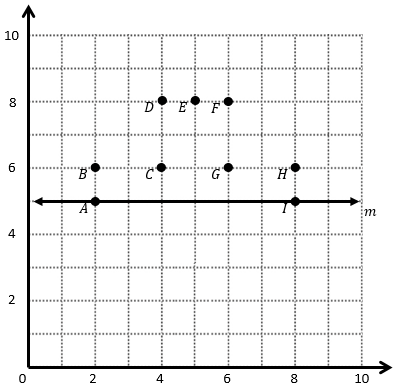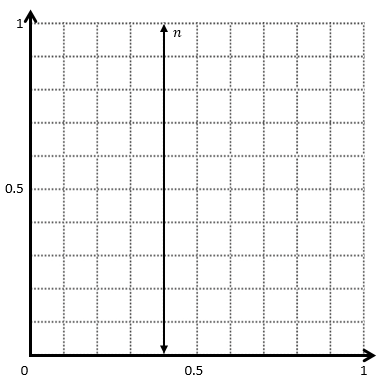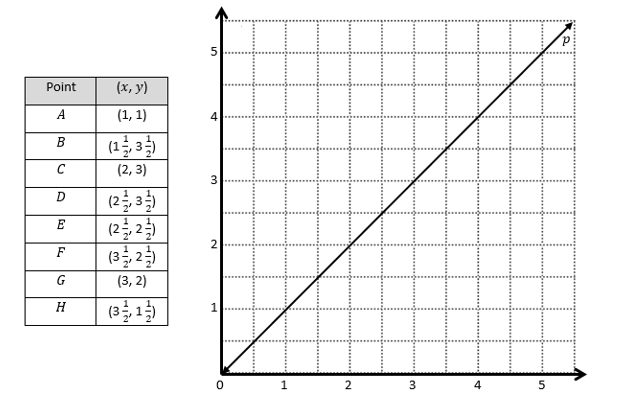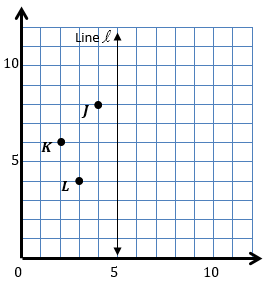# Patterns and the Coordinate Plane

## Objective

Draw symmetric figures on a coordinate plane.

## Common Core Standards

### Core Standards

?

• 5.G.A.1 — Use a pair of perpendicular number lines, called axes, to define a coordinate system, with the intersection of the lines (the origin) arranged to coincide with the 0 on each line and a given point in the plane located by using an ordered pair of numbers, called its coordinates. Understand that the first number indicates how far to travel from the origin in the direction of one axis, and the second number indicates how far to travel in the direction of the second axis, with the convention that the names of the two axes and the coordinates correspond (e.g., x-axis and x-coordinate, y-axis and y-coordinate).

• 5.G.A.2 — Represent real world and mathematical problems by graphing points in the first quadrant of the coordinate plane, and interpret coordinate values of points in the context of the situation.

?

• 4.G.A.3

## Criteria for Success

?

1. Given plotted points of half of a symmetric drawing on the coordinate plane and the line of symmetry, plot the other half of the figure.
2. Given coordinates of half of a symmetric drawing on the coordinate plane and the line of symmetry, plot the other half of the figure.
3. Understand that corresponding points in a symmetric figure are the same distance from the line of symmetry and, when connected by a line segment, form a perpendicular intersection with the line of symmetry.
4. Observe patterns in the coordinates of corresponding points across a horizontal or vertical line of symmetry.
5. Find all lines of symmetry in a symmetric figure on the coordinate plane.

## Tips for Teachers

?

The Progressions for the Common Core State Standards in Mathematics, Geometry, K–6 notes that “students solve mathematical and real-world problems using coordinates. For example, they plan to draw a symmetric figure using computer software in which students input coordinates that are then connected by line segments” (pp. 17–18). Thus, you may decide to make this lesson into one using computer software, such as modifying the Desmos activity, Blue Point Rule, to fit within the scope of 5.G.2. But, if computers are not available to the whole class, because there are no released items from the main standardized tests (PARCC, SBAC, NY Regents, MCAS), it’s at your discretion to keep this lesson in the unit.

#### Fishtank Plus

• Problem Set
• Student Handout Editor
• Vocabulary Package

?

### Problem 1

The Swiss flag is a perfect square with two lines of symmetry. One part of the Swiss flag, as well as one of its lines of symmetry, m, is shown on the coordinate grid below.What does the whole flag look like? What are the coordinates of the additional points you drew? Where is its other line of symmetry?

#### References

EngageNY Mathematics Grade 5 Mathematics > Module 6 > Topic D > Lesson 18Concept Development

Grade 5 Mathematics > Module 6 > Topic D > Lesson 18 of the New York State Common Core Mathematics Curriculum from EngageNY and Great Minds. © 2015 Great Minds. Licensed by EngageNY of the New York State Education Department under the CC BY-NC-SA 3.0 US license. Accessed Dec. 2, 2016, 5:15 p.m..

Modified by The Match Foundation, Inc.

### Problem 2

Given the following line of symmetry, n, construct a symmetric figure in the plane and record its coordinates below. Explain how you know it is symmetric.### Problem 3

1. Plot the points from the table on the grid. Then, draw line segments to connect the points in order.1. The points create half of a symmetric figure about line p. Create the other half of the figure and record the coordinates below.

#### References

EngageNY Mathematics Grade 5 Mathematics > Module 6 > Topic D > Lesson 18Concept Development

Grade 5 Mathematics > Module 6 > Topic D > Lesson 18 of the New York State Common Core Mathematics Curriculum from EngageNY and Great Minds. © 2015 Great Minds. Licensed by EngageNY of the New York State Education Department under the CC BY-NC-SA 3.0 US license. Accessed Dec. 2, 2016, 5:15 p.m..

Modified by The Match Foundation, Inc.

## Problem Set & Homework

#### Discussion of Problem Set

• Does the orientation of the line of symmetry change the way symmetric points must be drawn?  Why or why not?
• What must be true for a pair of points to be symmetric about a line?
• When drawing figures about a vertical line of symmetry on a coordinate plane, why does only the x-coordinate differ in symmetric coordinate pairs? What about with a vertical line of symmetry?
• In #3, did your partner plot any points on the line of symmetry?  If so, did the pattern of how the x- and y-coordinates change continue for these fixed points?

?

Use the coordinate plane below to answer the question.Points JK, and L, combined with additional points that are not shown, create a figure that is symmetric about the line l. What are the coordinates of the additional points?

?